## ISIS 2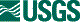ISIS Application Documentation

# equalizer

Printer Friendly View | TOC | Home

Tone matches map projected cubes

 Overview Parameters Example 1 Example 2 Example 3 Example 4 Example 5

## Description

This program tone matches or equalizes the brightness and/or contrast of a list of input cubes prior to mosaicking. All the input cubes, including mosaics, must be in identical map projection since equalizer uses the mapping information to gather statistics in overlapping areas.

Statistic gathering is done on a band-by-band basis. The statistics are used in a least squares solution to determine multiplicative (GAIN or MULT) and additive (OFFSET or BASE) corrections for each image. In addition to simply calculating corrective factors and applying said factors to each image (BOTH), the user has the option to only calculate statistics (CALCULATE) and then examine these gathered statistics in a text file (OUTSTATS). Then, equalizer can be run again using that statistics file as input (INSTATS) to apply correction to any or all of the images without the need to calculate corrective factors again.

Corrective factors will be undetermined if there are insufficient valid overlaps or if there are any images that have no overlaps. If an output statistics file is provided (OUTSTATS), images that do not overlap will appear in the file.

When corrective factors are undetermined, the user can use the RECALCULATE process option to try to solve them. To do this, images can be added to the input list to try to create more overlaps or images without overlaps can be removed. An input statistics file (INSTATS) containing previously calculated image statistics must be provided. These statistics will be reloaded so overlap statistics will only be recalculated for new images in the modified input list. If all the images have overlaps and sufficient valid overlaps exist, corrective factors will be determined. Statistics can be recalculated as many times as desired by repeating the above steps.

Additionally, the RETRYBOTH process option may be used to recalculate statistics and then apply corrective factors. This requires the user to provide an INSTATS file containing previously calculated statistics. It is also suggested that the user provide an OUTSTATS file in case corrective factors cannot be determined. If the corrective factors are determined, they will then be applied to the input images.

The addition of these RETRYBOTH and RECALCULATE parameters has modified the content of the output statistics. Additional information is included in the output statistics so that previously calculated overlap statistics can be reloaded when using these parameters. Note that statistics files created with previous versions of ISIS can still be used as input statistics.

The actual equation to be used for equalization, for ADJUST=BRIGHTNESS, CONTRAST or BOTH, on each band in each cube is as follows:

```        newdn(s,l,b)   =   (olddn(s,l,b) - avg(b)) * GAIN(b) + (avg(b) + OFFSET(b))
```
where
```        s = sample index
l = line index
b = band index
```
The actual equation to be used for equalization, for ADJUST=GAIN, on each band in each cube is
```        newdn(s,l,b)   =   olddn(s,l,b) * GAIN(b)
```
where
```        s = sample index
l = line index
b = band index
```

Prior to equalizing, the user can choose whether to adjust the brightness and/or contrast of the cubes. The default is to adjust both; however, if the brightness (average) of all the cubes is the same, then simply adjusting the contrast may suffice. Likewise, if the standard deviation of all the cubes is similar, then a contrast adjustment is not necessary. Adjusting for contrast only implies the OFFSET values will be held to zero. Similarly, adjusting for brightness implies the GAIN values will be held to one.

The OFFSET and GAIN values are computed independently for each image, therefore we have two least squares computations with N unknowns, where N is the number of cubes to be equalized. The overlaps, M, between all the cubes are computed, and in some cases M < N. This implies an underdetermined system, and the program will report an error if this occurs. You can hold one or more images to alleviate this problem. Holding an image forces GAIN and OFFSET to 1.0 and 0.0 for that image, respectively.

If the user chooses to apply correction to the images, then a list of output file names can be specified with the TOLIST parameter. If no TOLIST is specified, the equalized cubes will be named the same as the input cubes with the addition of '.equ' prior to the '.cub' extension, and placed in the same directories as their input files.

For the sake of efficiency, the user may choose to set the "sampling percent" to be less than its default value of 100.0. By doing so, the program will likely perform its statistic gathering noticeably faster, but at the risk of losing accuracy in the results. It should be noted that the user also runs the risk of encountering an error if decreasing the sampling percent results in the amount of valid data in the calculated overlaps being less than the minimum set by the MINCOUNT parameter (default value of 1000). Sampling percent must be a decimal value between 0.0 (exclusive) and 100.0 (inclusive).

This program defaults to solving the least-squares system using the SPARSE matrix method. This method is able to find valid solutions, even when no hold list is provided. The QRD method is fairly accurate and fast, but does not produce valid results in all cases, especially if no images are held. Previous versions of equalizer used this method to solve.

## Related Applications to Previous Versions of ISIS

This program replaces the following application existing in previous versions of ISIS:
• b4equal

## Parameter Groups

### Files

Name Description
FROMLIST List of cubes to equalize
HOLDLIST List of filenames to hold
TOLIST List of the equalized cubes to be created
OUTSTATS Output text file containing thorough equalization-related statistics
INSTATS Input text file containing thorough equalization-related statistics, used for applying correction

### ProcessingOptions

Name Description
PROCESS Calculate statistics, apply correction, or do both
SOLVEMETHOD The solve method to be used to calculate the equalization statistics

### CalculationOptions

Name Description
MINCOUNT Minimum number of points in overlapping area required to be used in the solution
WEIGHT Whether or not overlaps should be weighted
PERCENT Percentage of the lines to consider when gathering overall cube statistics and overlap statistics

### Files: FROMLIST

#### Description

A list of map projected cubes to equalize. The Mapping groups must match in order to do the equalization.

 Type filename input

### Files: HOLDLIST

#### Description

List of cubes that are to be held in the equalization. An additive and a multiplicative factor of 0 and 1 will be applied to all cubes that were held. All cubes listed in this file must also be contained in FROMLIST.

 Type filename input None *.txt *.lis *.lst

### Files: TOLIST

#### Description

This list of output files must contain a file name to correspond to each input file in the FROMLIST. Input files will be associated with output files by index, so that the equalized product of the first file in the FROMLIST will be written to the name and location of the first file in the TOLIST, and so on. Each output file will be written to the exact location with the exact name specified, unless the location and name happen to be identical to those of its corresponding input file, in which case an error will be thrown. If this list is not specified, but the APPLY option is still selected, the output files will be placed in the directories of their input files, and named the same with the exception of an added ".equ" extension (e.g., "foobar.cub" becomes "foobar.equ.cub").

 Type filename input Automatic

### Files: OUTSTATS

#### Description

This file will contain the statistics of all of the overlapping areas in every band along with the computed equalizing factors (OFFSET and GAIN). Specifying this output file is optional.

 Type filename output None *.txt *.pvl *.lis *.lst *.dat

### Files: INSTATS

#### Description

This file must contain the statistics of all the overlapping areas in every band along with the computed equalizing factors (OFFSET and GAIN) for every image in the FROMLIST.

 Type filename input *.txt *.pvl *.lis *.lst *.dat

### ProcessingOptions: PROCESS

#### Description

This option allows the user to decide whether they want to do the equalization calculations and then go on to apply correction afterwards, apply correction to the images off previously gathered statistics, or do the calculations but not apply correction. If the APPLY option is not selected, you must specify an OUTSTATS file. If it is selected, you can still optionally specify an OUTSTATS file and the GAIN/OFFSET values will be applied to each input cube. The names and locations of the output cubes are specified by the TOLIST file.

Type string
Default BOTH
Option List:
Option Brief Description
BOTH Calculate statistics and apply correction This option will calculate image statistics and then apply corrections from the results.

#### Exclusions

• INSTATS
RETRYBOTH Recalculate statistics and apply correction This option will load previously calculated image statistics, calculate statistics for any new images in FROMLIST and then apply corrections from the results (if the corrections can be determined).

• MINCOUNT
• WEIGHT
• PERCENT
• SOLVEMETHOD

#### Inclusions

• INSTATS
CALCULATE Calculate statistics only This option will calculate image statistics and write them to an output PVL file. Image corrections will not be applied.

• TOLIST
• INSTATS

#### Inclusions

• OUTSTATS
RECALCULATE Recalculate statistics using previously calculated statistics This option will load previously calculated image statistics, calculate statistics for any new images in FROMLIST and then write them to an output PVL file. Image corrections will not be applied.

• TOLIST
• MINCOUNT
• WEIGHT
• PERCENT
• SOLVEMETHOD

#### Inclusions

• INSTATS
• OUTSTATS
APPLY Apply correction only This option will use previously generated statistics to apply correction to the images.

• HOLDLIST
• OUTSTATS
• MINCOUNT
• WEIGHT
• PERCENT
• SOLVEMETHOD

• INSTATS

### ProcessingOptions: SOLVEMETHOD

#### Description

This option allows the user to choose the solve method for the least-squares system. The default is SPARSE.

Type string
Default SPARSE
Option List:
Option Brief Description
QRD The QR Decomposition method is least accurate when no hold list is provided. Solve the least-squares system using QR decomposition. This method is likely to result in invalid values when no hold list is provided.
SPARSE The SPARSE is the most accurate when no hold list is provided. Solve the least-squares system using a sparse matrix formulation of the LU decomposition. This is the best method to use if no hold list is provided.

#### Description

This option allows the user to select the algorithm that will be used to adjust the pixel values. The BRIGHTNESS mode will equalize using only an offset (the gain will be set to 1.0), and the CONTRAST mode will equalize using only a gain (the offset will be set to 0.0) but keeping the images around their current average. The GAIN option will equalize using only a gain and will not normalize the output values. The BOTH option will equalize using both an offset and a gain. In most cases this option will give you the best results.

Type string
Default BOTH
Option List:
Option Brief Description
BOTH Adjust the brightness and contrast of the images. This option will use an algorithm that equalizes both the brightness and contrast of the images. The equation this option uses is:
```                output = (input - average(input)) * gain + offset + average(input)
```
BRIGHTNESS Adjust the brightness of the images using calculated offsets This option will use an algorithm that only equalizes the brightness of the images by calculating the offset for each. This option should be used only if the variances/standard deviations of the images are close. The equation this option uses is:
```                output = offset + average(input)
```
CONTRAST Adjust the contrast of the images only using calculated gains This option will use an algorithm that only equalizes the contrast of the images by calculating the gain for each. This option should only be used if the brightnesses of the images are already close. The equation this option uses is:
```                output = input * gain + average(input) * gain
```
GAIN Adjust the contrast of the images without normalization This option will use an algorithm that only equalizes the contrast of the images by calculating the gain for each. No offsets will be applied; the resulting image will only have a gain adjustment and no normalization. This option should only be used if the brightnesses of the images are already close. The equation this option uses is:
```                output = input * gain
```

### CalculationOptions: MINCOUNT

#### Description

If the number of points in the overlapping area meets or exceeds this value, the area will go into the least-squares solution as a "known." Otherwise it will not be included in the calculations.

 Type integer 1000

### CalculationOptions: WEIGHT

#### Description

This option allows the user to decide whether they want to weight the least squares solution based on how large the overlap area is, or if they want no weighting at all. If this parameter is set to "true," then larger overlapping areas will have more of an impact on the corrective factors than smaller overlaps.

 Type boolean FALSE

### CalculationOptions: PERCENT

#### Description

The percentage of the lines in each area to consider in the process-by-line solutions for finding overall cube statistics and overlap statistics. This value must be a decimal value between 0.0 (exclusive) and 100.0 (inclusive).

 Type double 100.0 0.0 (exclusive) 100.0 (inclusive)

### Example 1

Calculate Statistics and Apply Correction

#### Description

This example shows the most typical use of the equalizer application. The defaults are to perform both calculation and adjustment for both the gain and offset.

#### Command Line

equalizer fromlist= FromList.lst holdlist= HoldList.lst
Specify a list of images to equalize along with a holding list that enables the necessary calculations to be performed. All other options are at default values.

#### GUI Screenshot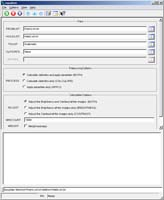Equalizer GUI Example GUI Screenshot of the GUI with parameters set to calculate the gain and offset and then to apply corrections. Because the TOLIST parameter is set to its default value of "Automatic," each output image will be placed into the directory of its corresponding input image. Also, because the OUTSTATS parameter is set to its default value of "None", a record of the calculations performed will only be written to the print file.

#### Input Image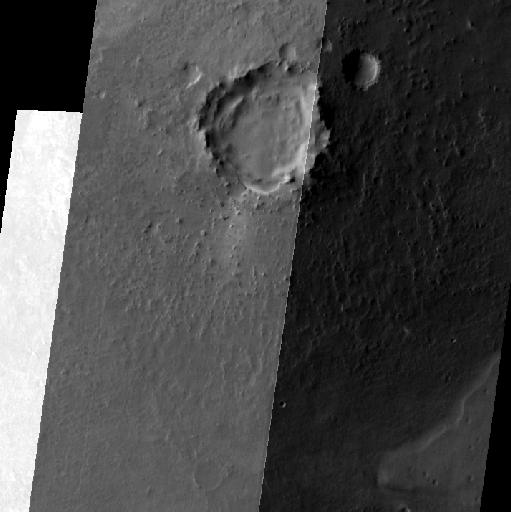Mosaic of unequalized input images Mosaic of the input images for equalizer Parameter Name: FROMLIST This is a small section of the input images for the equalizer example mosaicked together.

#### Data Files

Links open in a new window.
Click here to see the list of input images to be equalized This list contains the names of three files to calculate statistics upon, and then equalize. The file names are separated by new lines. This list contains the name of one file from the FROMLIST to be held during calculations. When multiple images are held, their file names are likewise separated by new lines.

#### Output Image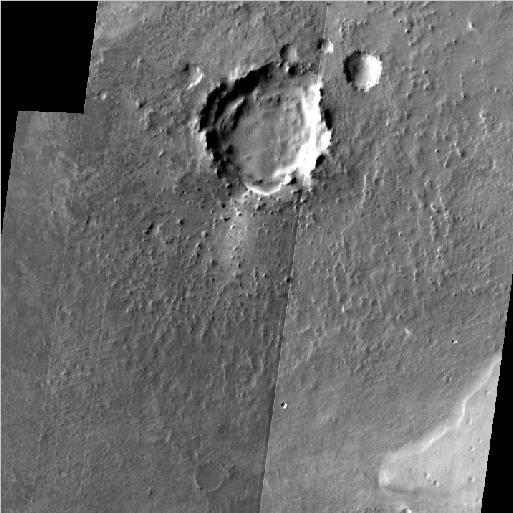Mosaic showing results of the equalizer application. Mosaic of the output images for equalizer Parameter Name: TOLIST This is a small section of the equalized output images mosaicked together.

### Example 2

Calculate Statistics

#### Description

This example shows how to use the equalizer application to calculate equalization statistics and write them to a file, without applying the corrections to the input images.

#### Command Line

equalizer fromlist= FromList.lst holdlist=/HoldList.lst outstats= stats.pvl process= calculate
In addition to specifying a fromlist and a holdlist, we must also specify a location to write the output statistics PVL file, and set the process type to "calculate." Since no output images will be created, we do not (and cannot), specify a tolist. All other options are at default values.

#### GUI Screenshot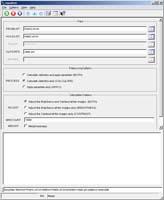Equalizer GUI Example GUI Screenshot of the GUI with parameters set to calculate equalization statistics on a set of input images.

#### Input ImageMosaic of unequalized input images. Mosaic of the input images for equalizer Parameter Name: FROMLIST This is a small section of the input images for the equalizer example mosaicked together.

#### Data Files

Links open in a new window.
Click here to see the list of images to calculate statistics upon This list contains the names of three files to calculate statistics upon. The file names are separated by new lines. This list contains the name of one file from the FROMLIST to be held during calculations. When multiple images are held, their file names are likewise separated by new lines. This PVL file contains thorough equalization-related statistics that can be used for applying correction to any or all images used to generate this file.

### Example 3

Apply Correction

#### Description

This example shows the use of the equalizer application for applying correction to a subset of the images used to produce a specified input statistics file.

#### Command Line

equalizer fromlist= Input.lst tolist= Output.lst instats= stats.pvl process= apply
Specify a fromlist that is a sublist of the fromlist from Example 2. Because calculations have already been performed, no holdlist is specified, and since we want to place the output images in a specific location with specific names, a tolist is specified. Finally, we set the value of the instats parameter to be the output statistics file generated in Example 2, and set the process to "apply." All other options are at default values.

#### GUI Screenshot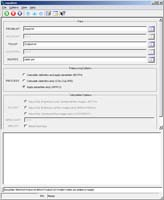Equalizer GUI Example GUI Screenshot of the GUI with parameters set to apply correction to a subset of the images used to produce a specified input statistics file.

#### Data Files

Links open in a new window.
Click here to see the list of images to be equalized This list contains the names of two files to equalize. Both file names appear in the FROMLIST file from the previous example, and both file names have calculated gain and offset coefficients calculated for them in the INSTATS file. Since we do not wish to create a new file for the held image, however, we chose not to include it in this new list. The file names are separated by new lines. This list contains the names of two files to be written as outputs from the equalization process. The index of each file name corresponds to the index of a file from this example's FROMLIST file. Here, the output images retain their original names, but are written to the current directory. This PVL file contains thorough equalization-related statistics for applying correction to any or all images used to generate this file. In this example, two of the three files used to generate these statistics have been selected for equalization.

#### Output ImageMosaic showing results of the equalizer application. Mosaic of the output images for equalizer Parameter Name: TOLIST This is a small section of the equalized output images and the held image from Example 2 mosaicked together. Note that since we only chose to omit the held image from Example 2 when applying correction, that the results are the same as those in Example 1.

### Example 4

Recalculate Statistics

#### Description

This example shows the use of the equalizer application for recalculating statistics after initially calculating statistics where some images do not have any overlaps.

#### Command Line

equalizer fromlist=fixedList.lst instats=nonOverlapStats.pvl outstats=fixedStats.pvl process=recalculate
In addition to specifying a fromlist, we must also specify the previously calculated statistics PVL file (instats), specify a location to write the output statistics PVL file (outstats), and set the process type to "recalculate." Since no output images will be created, we do not (and cannot), specify a tolist. All other options are at default values.

#### GUI Screenshot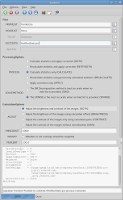Equalizer GUI Example GUI Screenshot of the GUI with parameters set to initially calculate statistics for a set of images with non-overlapping images.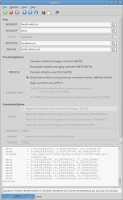Equalizer GUI Example GUI Screenshot of the GUI with parameters set to recalculate statistics after calculating statistics for a set of images with non-overlapping images.

#### Input Images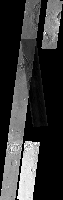Mosaic of unequalized input images where two images do not have overlaps Mosaic of the input images for equalizer for initial calculation This is a small section of the input images mosaicked together. Two of the images do not have any overlaps.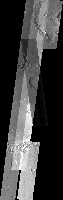Mosaic of unequalized input images where all images have valid overlaps Mosaic of the input images for equalizer for recalculation Parameter Name: FROMLIST This is a small section of the input images mosaicked together. The non-overlaps have been corrected.

#### Data Files

Links open in a new window.
 Click here to see the list with non-overlapping images used to calculate statistics This list contains the names of four files where two images do not have any overlaps. This list contains the names of five files that all have valid overlaps. The third file, I50695002EDR.proj.reduced.cub, was added to fix the non-overlapping areas. (FROMLIST) This PVL file contains equalization-related statistics where corrective factors have not been determined (since there were images without overlaps). This can be used to recalculate statistics for a corrected input image list. Overlap statistics will only be calculated for new overlaps. (INSTATS) This PVL file contains equalization-related statistics that were recalculated from a previous run of equalizer. This can be used for applying correction to any or all images used to generate this file. (OUTSTATS)

### Example 5

Recalculate Statistics and Apply Correction (RETRYBOTH)

#### Description

This example shows the use of the equalizer application for recalculating statistics after initially trying to calculate and apply corrections on a non-overlapping data set.

#### Command Line

equalizer fromlist=fixedFromlist.lis tolist=tolist.lis outstats=retryBothStats.pvl instats=nonOverlapStats.pvl process=retryboth
In addition to specifying a fromlist, we must also specify the previously calculated statistics (instats). We should also provide a location to write the output statistics PVL file (outstats) if we still have images without overlaps in our fromlist. We will also provide a list of output files (tolist).

#### GUI Screenshot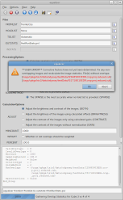Equalizer GUI Example GUI Screenshot of the GUI with parameters set to calculate statistics and apply corrections (BOTH). This fails because some of the images do not have any overlaps (see the application log at the bottom of the screenshot).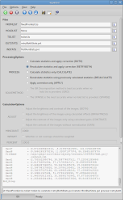Equalizer GUI Example GUI Screenshot of the GUI with parameters set to recalculate statistics from previously calculated statistics and apply corrections (RETRYBOTH) to the input images.

#### Input ImagesMosaic of unequalized input images where two images do not have overlaps Mosaic of the input images for equalizer for initial calculation This is a small section of the input images mosaicked together. Two of the images do not have any overlaps.Mosaic of unequalized input images where all images have valid overlaps Mosaic of the input images for equalizer before corrections are applied Parameter Name: FROMLIST This is a small section of the input images mosaicked together. The non-overlaps have been corrected by adding I50695002EDR.proj.reduced.cub to the input list.

#### Data Files

Links open in a new window.
 Click here to see the corrected list with all overlapping images used to recalculate statistics This list contains the names of five files that all have valid overlaps. The third file, I50695002EDR.proj.reduced.cub, was added to fix non-overlapping areas from a previous run. (FROMLIST) This list contains the names of the five equalized output files that will be created. (TOLIST) This PVL file contains equalization-related statistics where corrective factors have not been determined (since there were images without overlaps). This can be used to recalculate statistics for a corrected input image list. Overlap statistics will only be calculated for new overlaps. (INSTATS) This PVL file contains equalization-related statistics that were recalculated from a previous run of equalizer. (OUTSTATS)

#### Output Image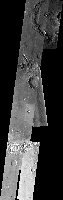Mosaic of equalized output images Mosaic of the equalized output images Parameter Name: TOLIST This is a small section of the equalized output images mosaicked together.